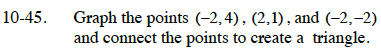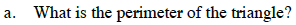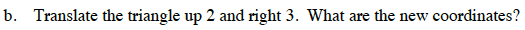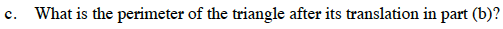Home > ACC7 > Chapter cc310 > Lesson cc310.1.3 > Problem10-45

10-45.
1. Graph the points (−2, 4), (2, 1), (−2, −2) and connect the points to create a triangle. 10-45 HW eTool (Desmos). Homework Help ✎

1. What is the perimeter of the triangle?

2. Translate the triangle up 2 and right 3. What are the new coordinates?

3. What is the perimeter of the triangle after its translation in part (b)?Divide the triangle into two right triangles. Then use the graph to find some of the missing lengths.

Notice that the two triangles are congruent. Find the hypotenuse of one of the triangles and that length will also be the length of the other hypotenuse. Use the Pythagorean Theorem.

Now add up all of the sides to get the perimeter.

3² + 4² = hypotenuse²
25 = hypotenuse²

$\sqrt{25}=\sqrt{\text{hypotenuse}}$

hypotenuse = 5(1, 6), (5, 3), and (1, 0)Does a rigid transformation such as a translation change the size of the shape?

Use the eTool below to graph the points and create a triangle.
Click the link at right for the full version of the eTool: CC3 10-45 HW eTool.